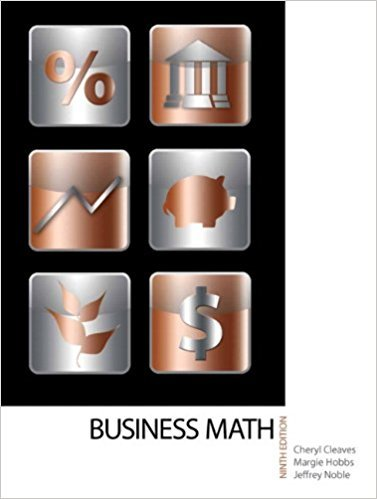×
×

# Use the last-in, first-out method to find the cost of goods sold and the cost of endingISBN: 9780135108178 355

## Solution for problem 7 Chapter 18

• Textbook Solutions
• 2901 Step-by-step solutions solved by professors and subject experts
• Get 24/7 help from StudySoup virtual teaching assistants4 5 1 422 Reviews
14
2
Problem 7

Use the last-in, first-out method to find the cost of goods sold and the cost of ending inventory using the table from Exercise 1 and the fact that the ending inventory is 200 units.

Step-by-Step Solution:
Step 1 of 3

K-2 CCSS Strategies Add To: Result Unknown Level 1: Count All 5 + 4 = IIIII IIII Level 2: Counting On Say 5 and then, using your fingers to model, 6, 7, 8, 9 Level 3: Doubles Plus/Minus 5 + 4= 5+ 4= 1 + 4 +4 = 1 + 8 = 9 Add To: Change Unknown Level 1: N/A Level 2: Counting On 5 + = 9 Say 5, and then, using your fingers to model, 6, 7, 8, 9 Level 3: Rearrange 5 + = 9  9...

Step 2 of 3

Step 3 of 3

##### ISBN: 9780135108178

Unlock Textbook Solution